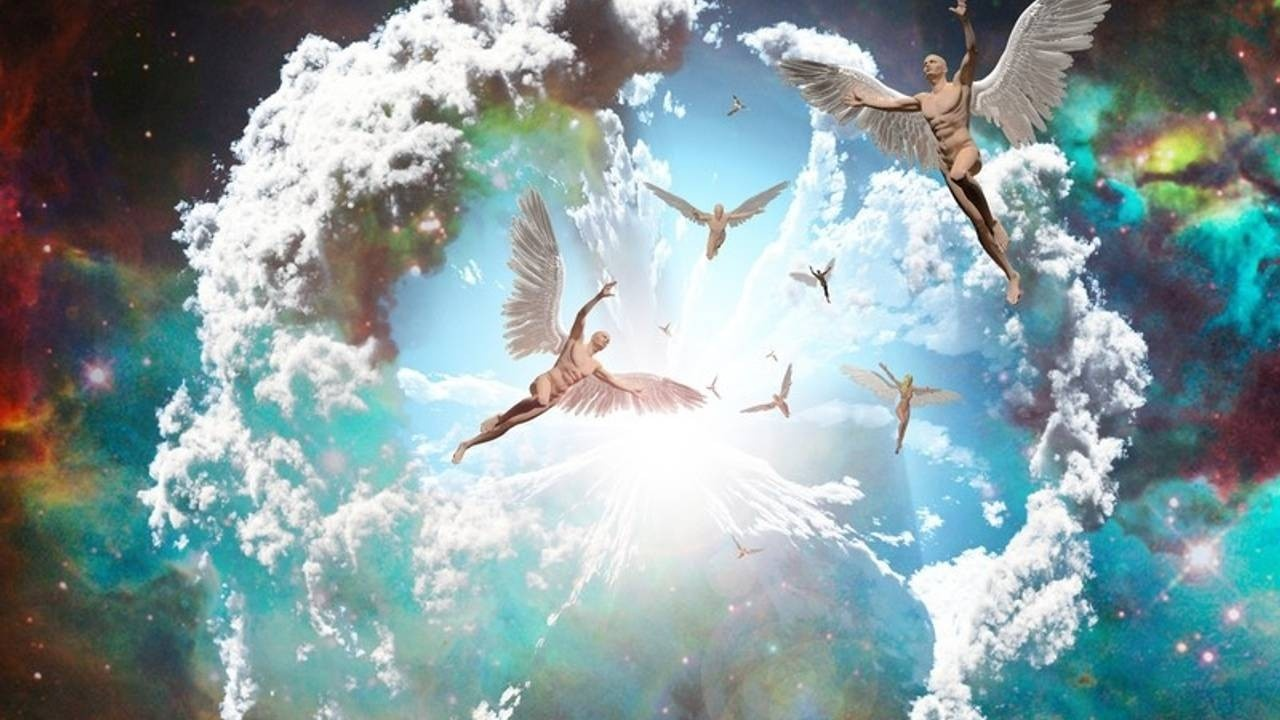# Latest News

## The 444 Energies of April 2021April 2021 is a another special month in so many ways ... the first one being that it is a "9" Universal Month.

We work out the "Universal Month" number by adding the month to the year. So

4+2+0+2+1=9

During a 9 Universal month we get lots of powerful numerology happening EVERY day because the vibration of the day will always match the number of the day itself.

For example on April 3rd:

3+(4+2+0+2+1) = 12 and 1+2=3 so we have a double 3 code.

Wheras on April 4th (4/4):

4+(4+2+0+2+1) = 13 and 1+3= 4 so it's a TRIPLE 4 code.

The 444 code also activates on the 13th and 22nd.

## Master Number 44 (444)

The Master numbers hold powerful codes for our evolution.

Many are "seeing" these numbers on clocks or number plates. 11:11, 222 etc.

The search for meaning can lead us to the angel number interpretations brought through by several channels/teachers.

For example in "angel numbers" 444 is a code often associated with the angelic realm itself.

But as WE...